# 3.5 Transformation of functions  (Page 11/21)

 Page 11 / 21

When examining the formula of a function that is the result of multiple transformations, how can you tell a horizontal stretch from a vertical stretch?

When examining the formula of a function that is the result of multiple transformations, how can you tell a horizontal compression from a vertical compression?

A horizontal compression results when a constant greater than 1 is multiplied by the input. A vertical compression results when a constant between 0 and 1 is multiplied by the output.

When examining the formula of a function that is the result of multiple transformations, how can you tell a reflection with respect to the x -axis from a reflection with respect to the y -axis?

How can you determine whether a function is odd or even from the formula of the function?

For a function $\text{\hspace{0.17em}}f,\text{\hspace{0.17em}}$ substitute $\text{\hspace{0.17em}}\left(-x\right)\text{\hspace{0.17em}}$ for $\text{\hspace{0.17em}}\left(x\right)\text{\hspace{0.17em}}$ in $\text{\hspace{0.17em}}f\left(x\right).\text{\hspace{0.17em}}$ Simplify. If the resulting function is the same as the original function, $\text{\hspace{0.17em}}f\left(-x\right)=f\left(x\right),\text{\hspace{0.17em}}$ then the function is even. If the resulting function is the opposite of the original function, $\text{\hspace{0.17em}}f\left(-x\right)=-f\left(x\right),\text{\hspace{0.17em}}$ then the original function is odd. If the function is not the same or the opposite, then the function is neither odd nor even.

## Algebraic

For the following exercises, write a formula for the function obtained when the graph is shifted as described.

$\text{\hspace{0.17em}}f\left(x\right)=\sqrt{x}\text{\hspace{0.17em}}$ is shifted up 1 unit and to the left 2 units.

$\text{\hspace{0.17em}}f\left(x\right)=|x|\text{\hspace{0.17em}}$ is shifted down 3 units and to the right 1 unit.

$g\left(x\right)=|x-1|-3$

$\text{\hspace{0.17em}}f\left(x\right)=\frac{1}{x}\text{\hspace{0.17em}}$ is shifted down 4 units and to the right 3 units.

$\text{\hspace{0.17em}}f\left(x\right)=\frac{1}{{x}^{2}}\text{\hspace{0.17em}}$ is shifted up 2 units and to the left 4 units.

$g\left(x\right)=\frac{1}{{\left(x+4\right)}^{2}}+2$

For the following exercises, describe how the graph of the function is a transformation of the graph of the original function $\text{\hspace{0.17em}}f.$

$y=f\left(x-49\right)$

$y=f\left(x+43\right)$

The graph of $\text{\hspace{0.17em}}f\left(x+43\right)\text{\hspace{0.17em}}$ is a horizontal shift to the left 43 units of the graph of $\text{\hspace{0.17em}}f.$

$y=f\left(x+3\right)$

$y=f\left(x-4\right)$

The graph of $\text{\hspace{0.17em}}f\left(x-4\right)\text{\hspace{0.17em}}$ is a horizontal shift to the right 4 units of the graph of $\text{\hspace{0.17em}}f.$

$y=f\left(x\right)+5$

$y=f\left(x\right)+8$

The graph of $\text{\hspace{0.17em}}f\left(x\right)+8\text{\hspace{0.17em}}$ is a vertical shift up 8 units of the graph of $\text{\hspace{0.17em}}f.$

$y=f\left(x\right)-2$

$y=f\left(x\right)-7$

The graph of $\text{\hspace{0.17em}}f\left(x\right)-7\text{\hspace{0.17em}}$ is a vertical shift down 7 units of the graph of $\text{\hspace{0.17em}}f.$

$y=f\left(x-2\right)+3$

$y=f\left(x+4\right)-1$

The graph of $f\left(x+4\right)-1$ is a horizontal shift to the left 4 units and a vertical shift down 1 unit of the graph of $f.$

For the following exercises, determine the interval(s) on which the function is increasing and decreasing.

$f\left(x\right)=4{\left(x+1\right)}^{2}-5$

$g\left(x\right)=5{\left(x+3\right)}^{2}-2$

decreasing on $\text{\hspace{0.17em}}\left(-\infty ,-3\right)\text{\hspace{0.17em}}$ and increasing on $\text{\hspace{0.17em}}\left(-3,\infty \right)$

$a\left(x\right)=\sqrt{-x+4}$

$k\left(x\right)=-3\sqrt{x}-1$

decreasing on $\left(0,\text{\hspace{0.17em}}\infty \right)$

## Graphical

For the following exercises, use the graph of $\text{\hspace{0.17em}}f\left(x\right)={2}^{x}\text{\hspace{0.17em}}$ shown in [link] to sketch a graph of each transformation of $\text{\hspace{0.17em}}f\left(x\right).$

$g\left(x\right)={2}^{x}+1$

$h\left(x\right)={2}^{x}-3$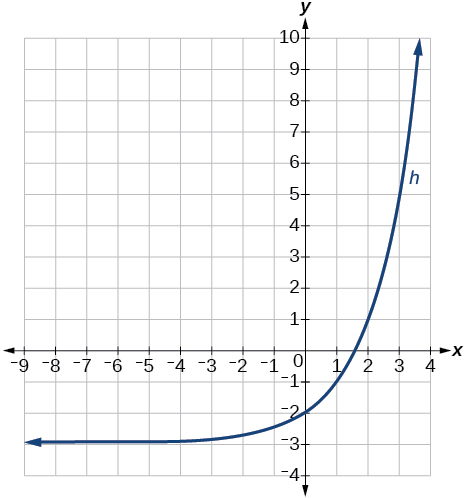$w\left(x\right)={2}^{x-1}$

For the following exercises, sketch a graph of the function as a transformation of the graph of one of the toolkit functions.

$f\left(t\right)={\left(t+1\right)}^{2}-3$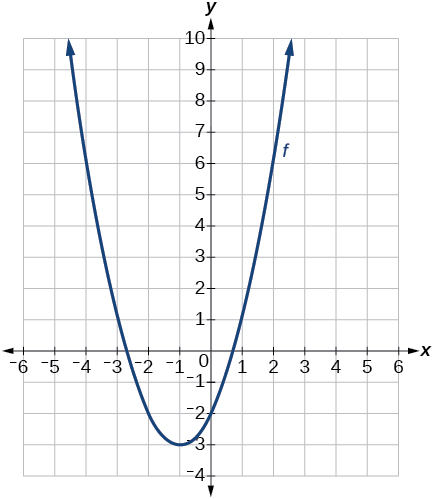$h\left(x\right)=|x-1|+4$

$k\left(x\right)={\left(x-2\right)}^{3}-1$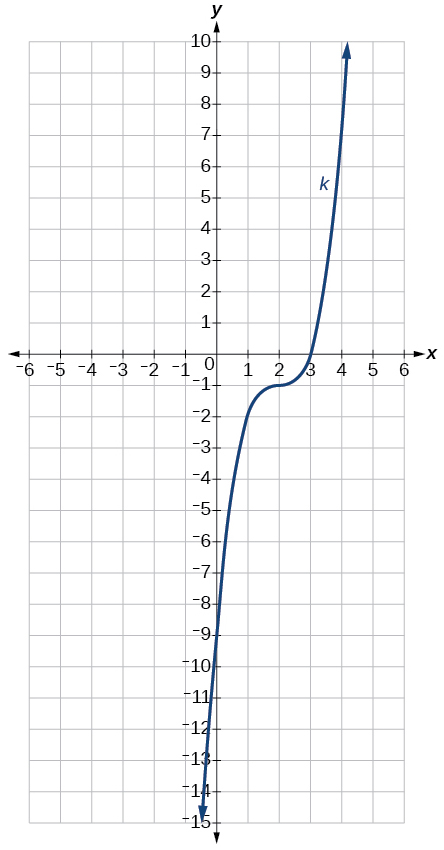$m\left(t\right)=3+\sqrt{t+2}$

## Numeric

Tabular representations for the functions $\text{\hspace{0.17em}}f,\text{\hspace{0.17em}}g,\text{\hspace{0.17em}}$ and $\text{\hspace{0.17em}}h\text{\hspace{0.17em}}$ are given below. Write $\text{\hspace{0.17em}}g\left(x\right)\text{\hspace{0.17em}}$ and $\text{\hspace{0.17em}}h\left(x\right)\text{\hspace{0.17em}}$ as transformations of $\text{\hspace{0.17em}}f\left(x\right).$

 $x$ −2 −1 0 1 2 $f\left(x\right)$ −2 −1 −3 1 2
 $x$ −1 0 1 2 3 $g\left(x\right)$ −2 −1 −3 1 2
 $x$ −2 −1 0 1 2 $h\left(x\right)$ −1 0 −2 2 3

$g\left(x\right)=f\left(x-1\right),\text{\hspace{0.17em}}h\left(x\right)=f\left(x\right)+1$

Tabular representations for the functions $\text{\hspace{0.17em}}f,\text{\hspace{0.17em}}g,\text{\hspace{0.17em}}$ and $\text{\hspace{0.17em}}h\text{\hspace{0.17em}}$ are given below. Write $\text{\hspace{0.17em}}g\left(x\right)\text{\hspace{0.17em}}$ and $\text{\hspace{0.17em}}h\left(x\right)\text{\hspace{0.17em}}$ as transformations of $\text{\hspace{0.17em}}f\left(x\right).$

 $x$ −2 −1 0 1 2 $f\left(x\right)$ −1 −3 4 2 1
 $x$ −3 −2 −1 0 1 $g\left(x\right)$ −1 −3 4 2 1
 $x$ −2 −1 0 1 2 $h\left(x\right)$ −2 −4 3 1 0

For the following exercises, write an equation for each graphed function by using transformations of the graphs of one of the toolkit functions.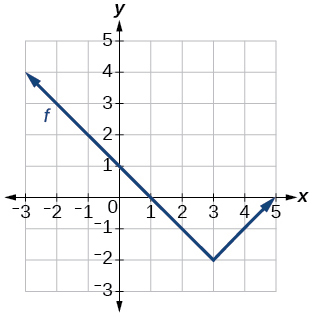$f\left(x\right)=|x-3|-2$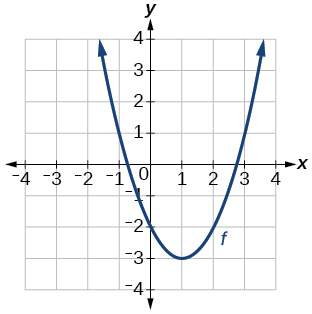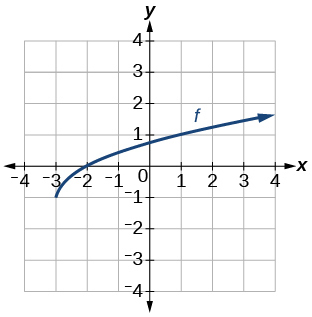$f\left(x\right)=\sqrt{x+3}-1$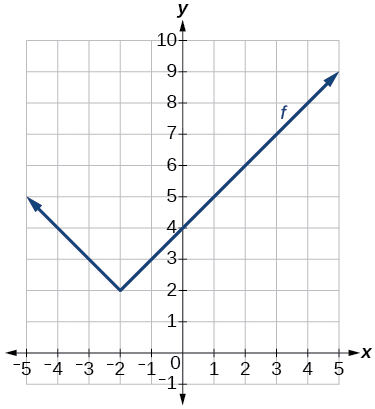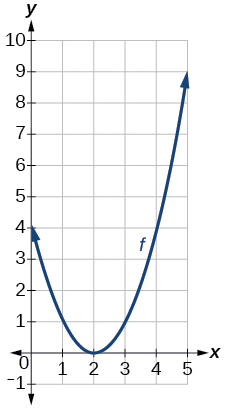$f\left(x\right)={\left(x-2\right)}^{2}$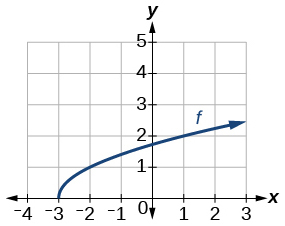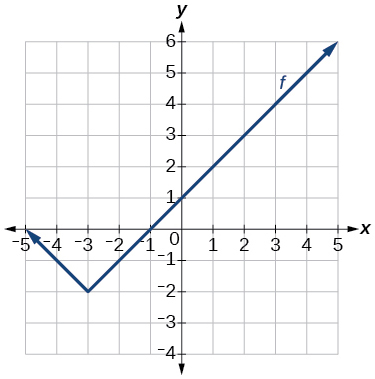$f\left(x\right)=|x+3|-2$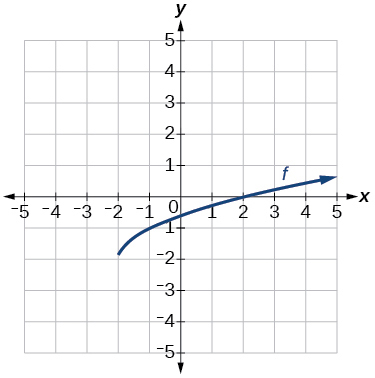For the following exercises, use the graphs of transformations of the square root function to find a formula for each of the functions.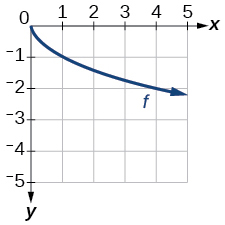$f\left(x\right)=-\sqrt{x}$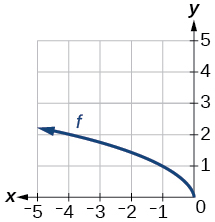For the following exercises, use the graphs of the transformed toolkit functions to write a formula for each of the resulting functions.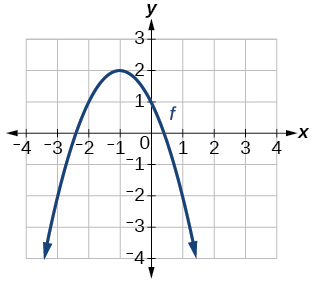$f\left(x\right)=-{\left(x+1\right)}^{2}+2$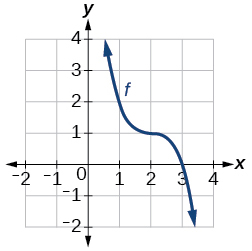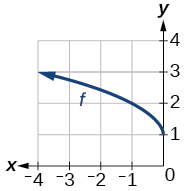$f\left(x\right)=\sqrt{-x}+1$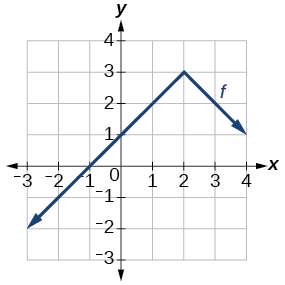For the following exercises, determine whether the function is odd, even, or neither.

$f\left(x\right)=3{x}^{4}$

even

$g\left(x\right)=\sqrt{x}$

$h\left(x\right)=\frac{1}{x}+3x$

odd

$f\left(x\right)={\left(x-2\right)}^{2}$

$g\left(x\right)=2{x}^{4}$

even

$h\left(x\right)=2x-{x}^{3}$

For the following exercises, describe how the graph of each function is a transformation of the graph of the original function $\text{\hspace{0.17em}}f.$

$g\left(x\right)=-f\left(x\right)$

The graph of $\text{\hspace{0.17em}}g\text{\hspace{0.17em}}$ is a vertical reflection (across the $\text{\hspace{0.17em}}x$ -axis) of the graph of $\text{\hspace{0.17em}}f.$

$g\left(x\right)=f\left(-x\right)$

$g\left(x\right)=4f\left(x\right)$

The graph of $\text{\hspace{0.17em}}g\text{\hspace{0.17em}}$ is a vertical stretch by a factor of 4 of the graph of $\text{\hspace{0.17em}}f.$

$g\left(x\right)=6f\left(x\right)$

$g\left(x\right)=f\left(5x\right)$

The graph of $\text{\hspace{0.17em}}g\text{\hspace{0.17em}}$ is a horizontal compression by a factor of $\text{\hspace{0.17em}}\frac{1}{5}\text{\hspace{0.17em}}$ of the graph of $\text{\hspace{0.17em}}f.$

$g\left(x\right)=f\left(2x\right)$

$g\left(x\right)=f\left(\frac{1}{3}x\right)$

The graph of $\text{\hspace{0.17em}}g\text{\hspace{0.17em}}$ is a horizontal stretch by a factor of 3 of the graph of $\text{\hspace{0.17em}}f.$

$g\left(x\right)=f\left(\frac{1}{5}x\right)$

$g\left(x\right)=3f\left(-x\right)$

The graph of $\text{\hspace{0.17em}}g\text{\hspace{0.17em}}$ is a horizontal reflection across the $\text{\hspace{0.17em}}y$ -axis and a vertical stretch by a factor of 3 of the graph of $\text{\hspace{0.17em}}f.$

$g\left(x\right)=-f\left(3x\right)$

For the following exercises, write a formula for the function $\text{\hspace{0.17em}}g\text{\hspace{0.17em}}$ that results when the graph of a given toolkit function is transformed as described.

The graph of $\text{\hspace{0.17em}}f\left(x\right)=|x|\text{\hspace{0.17em}}$ is reflected over the $\text{\hspace{0.17em}}y$ - axis and horizontally compressed by a factor of $\text{\hspace{0.17em}}\frac{1}{4}$ .

$g\left(x\right)=|-4x|$

The graph of $\text{\hspace{0.17em}}f\left(x\right)=\sqrt{x}\text{\hspace{0.17em}}$ is reflected over the $\text{\hspace{0.17em}}x$ -axis and horizontally stretched by a factor of 2.

The graph of $\text{\hspace{0.17em}}f\left(x\right)=\frac{1}{{x}^{2}}\text{\hspace{0.17em}}$ is vertically compressed by a factor of $\text{\hspace{0.17em}}\frac{1}{3},\text{\hspace{0.17em}}$ then shifted to the left 2 units and down 3 units.

$g\left(x\right)=\frac{1}{3{\left(x+2\right)}^{2}}-3$

The graph of $\text{\hspace{0.17em}}f\left(x\right)=\frac{1}{x}\text{\hspace{0.17em}}$ is vertically stretched by a factor of 8, then shifted to the right 4 units and up 2 units.

The graph of $\text{\hspace{0.17em}}f\left(x\right)={x}^{2}\text{\hspace{0.17em}}$ is vertically compressed by a factor of $\text{\hspace{0.17em}}\frac{1}{2},\text{\hspace{0.17em}}$ then shifted to the right 5 units and up 1 unit.

$g\left(x\right)=\frac{1}{2}{\left(x-5\right)}^{2}+1$

The graph of $\text{\hspace{0.17em}}f\left(x\right)={x}^{2}\text{\hspace{0.17em}}$ is horizontally stretched by a factor of 3, then shifted to the left 4 units and down 3 units.

For the following exercises, describe how the formula is a transformation of a toolkit function. Then sketch a graph of the transformation.

$g\left(x\right)=4{\left(x+1\right)}^{2}-5$

The graph of the function $\text{\hspace{0.17em}}f\left(x\right)={x}^{2}\text{\hspace{0.17em}}$ is shifted to the left 1 unit, stretched vertically by a factor of 4, and shifted down 5 units.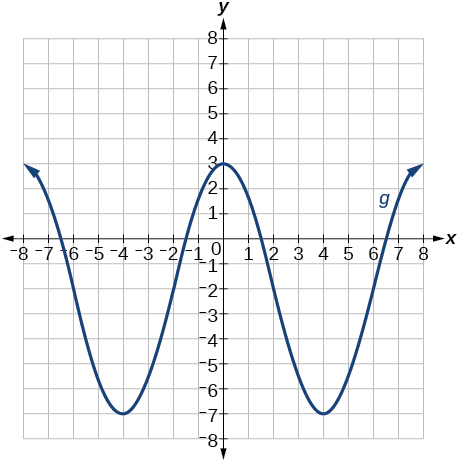$g\left(x\right)=5{\left(x+3\right)}^{2}-2$

$h\left(x\right)=-2|x-4|+3$

The graph of $\text{\hspace{0.17em}}f\left(x\right)=|x|\text{\hspace{0.17em}}$ is stretched vertically by a factor of 2, shifted horizontally 4 units to the right, reflected across the horizontal axis, and then shifted vertically 3 units up.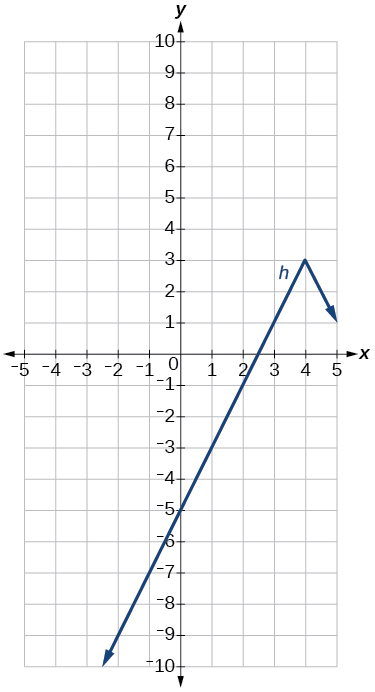$k\left(x\right)=-3\sqrt{x}-1$

$m\left(x\right)=\frac{1}{2}{x}^{3}$

The graph of the function $\text{\hspace{0.17em}}f\left(x\right)={x}^{3}\text{\hspace{0.17em}}$ is compressed vertically by a factor of $\text{\hspace{0.17em}}\frac{1}{2}.$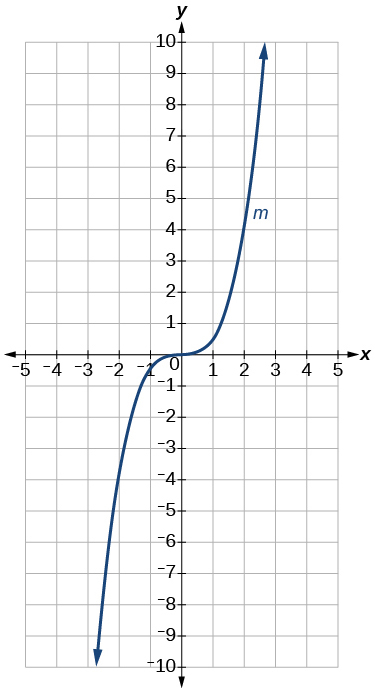$n\left(x\right)=\frac{1}{3}|x-2|$

$p\left(x\right)={\left(\frac{1}{3}x\right)}^{3}-3$

The graph of the function is stretched horizontally by a factor of 3 and then shifted vertically downward by 3 units.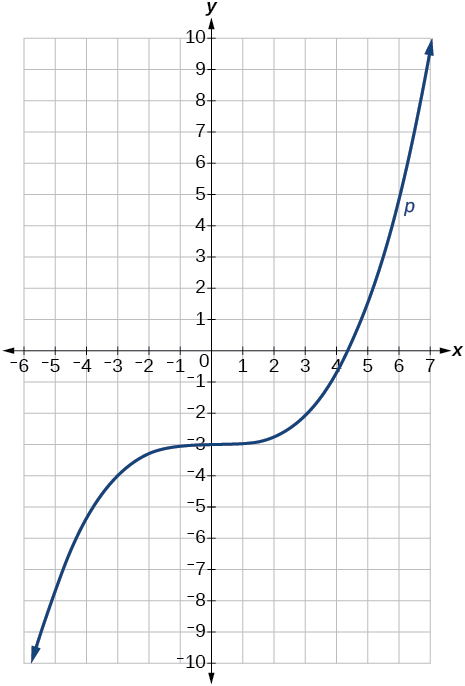$q\left(x\right)={\left(\frac{1}{4}x\right)}^{3}+1$

$a\left(x\right)=\sqrt{-x+4}$

The graph of $\text{\hspace{0.17em}}f\left(x\right)=\sqrt{x}\text{\hspace{0.17em}}$ is shifted right 4 units and then reflected across the vertical line $\text{\hspace{0.17em}}x=4.$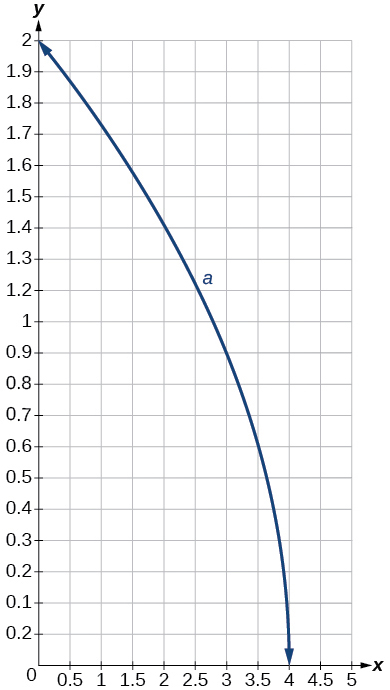For the following exercises, use the graph in [link] to sketch the given transformations.

$g\left(x\right)=f\left(x\right)-2$

$g\left(x\right)=-f\left(x\right)$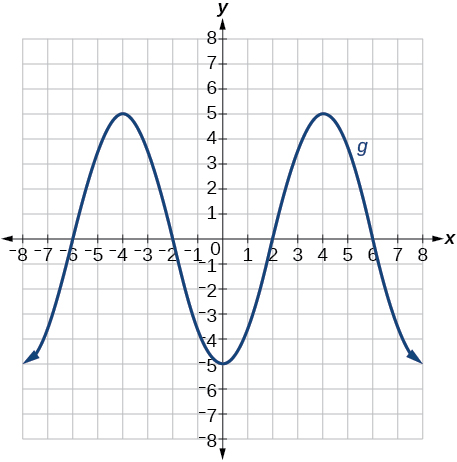$g\left(x\right)=f\left(x+1\right)$

$g\left(x\right)=f\left(x-2\right)$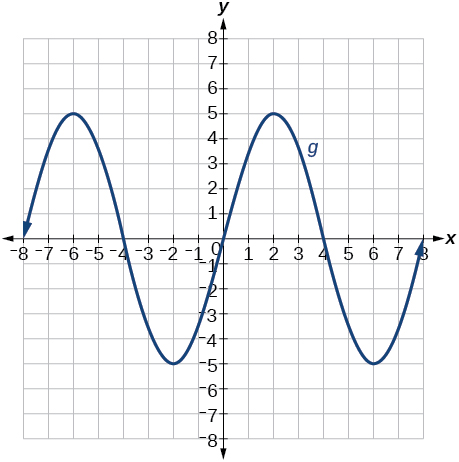#### Questions & Answers

x exposant 4 + 4 x exposant 3 + 8 exposant 2 + 4 x + 1 = 0
x exposent4+4x exposent3+8x exposent2+4x+1=0
HERVE
How can I solve for a domain and a codomains in a given function?
ranges
EDWIN
Thank you I mean range sir.
Oliver
proof for set theory
don't you know?
Inkoom
find to nearest one decimal place of centimeter the length of an arc of circle of radius length 12.5cm and subtending of centeral angle 1.6rad
factoring polynomial
find general solution of the Tanx=-1/root3,secx=2/root3
find general solution of the following equation
Nani
the value of 2 sin square 60 Cos 60
0.75
Lynne
0.75
Inkoom
when can I use sin, cos tan in a giving question
depending on the question
Nicholas
I am a carpenter and I have to cut and assemble a conventional roof line for a new home. The dimensions are: width 30'6" length 40'6". I want a 6 and 12 pitch. The roof is a full hip construction. Give me the L,W and height of rafters for the hip, hip jacks also the length of common jacks.
John
I want to learn the calculations
where can I get indices
I need matrices
Nasasira
hi
Raihany
Hi
Solomon
need help
Raihany
maybe provide us videos
Nasasira
Raihany
Hello
Cromwell
a
Amie
What do you mean by a
Cromwell
nothing. I accidentally press it
Amie
you guys know any app with matrices?
Khay
Ok
Cromwell
Solve the x? x=18+(24-3)=72
x-39=72 x=111
Suraj
Solve the formula for the indicated variable P=b+4a+2c, for b
Need help with this question please
b=-4ac-2c+P
Denisse
b=p-4a-2c
Suddhen
b= p - 4a - 2c
Snr
p=2(2a+C)+b
Suraj
b=p-2(2a+c)
Tapiwa
P=4a+b+2C
COLEMAN
b=P-4a-2c
COLEMAN
like Deadra, show me the step by step order of operation to alive for b
John
A laser rangefinder is locked on a comet approaching Earth. The distance g(x), in kilometers, of the comet after x days, for x in the interval 0 to 30 days, is given by g(x)=250,000csc(π30x). Graph g(x) on the interval [0, 35]. Evaluate g(5)  and interpret the information. What is the minimum distance between the comet and Earth? When does this occur? To which constant in the equation does this correspond? Find and discuss the meaning of any vertical asymptotes.
The sequence is {1,-1,1-1.....} hasByByByByBy David Martin